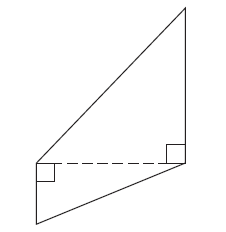Alyssa Z.

# Find the area in square inches of the figure shown.

Find the area in square inches of the figure shown.8 in.

17 in.

39 in.

36 in.

A four-sided figure is formed from two right triangles that share a leg, shown by a dashed line segment. The unshared leg and hypotenuse of one triangle are longer than those of the other triangle. The figure has four labeled lengths.

1. The larger triangle has the following lengths.
2. The unshared leg is labeled 36 inches.
3. The side opposite the right angle is labeled 39 inches.
4. The smaller triangle has the following lengths.
5. The unshared leg is labeled 8 inches.
6. The side opposite the right angle is labeled 17 inches.

in2David W.

tutor
That's a lot of work you're asking us to do for you. You know how to find the area of a triangle and you should know the Pythagorean theorem, which'll help you find the dotted length.
Report

23d

By:

Tutor
5 (19)

BS Mathematics, MD

## Still looking for help? Get the right answer, fast.

Get a free answer to a quick problem.
Most questions answered within 4 hours.

#### OR

Choose an expert and meet online. No packages or subscriptions, pay only for the time you need.